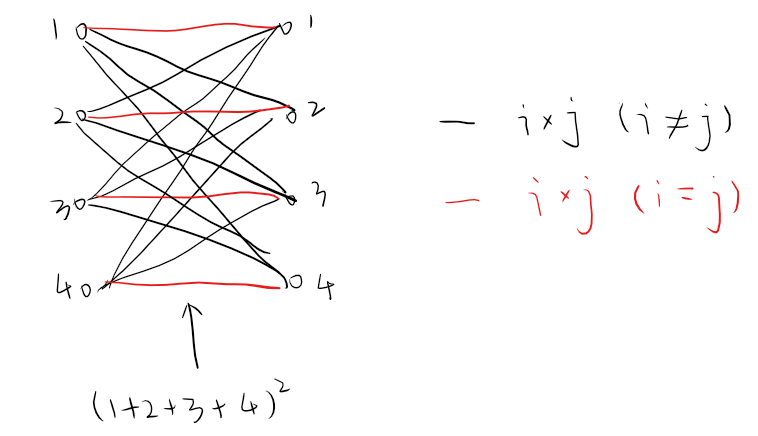# 题面

## #6. Sum square difference

The sum of the squares of the first ten natural numbers is,

The square of the sum of the first ten natural numbers is,

Hence the difference between the sum of the squares of the first ten natural numbers and the square of the sum is .

Find the difference between the sum of the squares of the first one hundred natural numbers and the square of the sum.

## #7. 10001st prime

By listing the first six prime numbers: 2, 3, 5, 7, 11, and 13, we can see that the 6th prime is 13.

What is the 10 001st prime number?

## #9. Special Pythagorean triplet

A Pythagorean triplet is a set of three natural numbers, , for which,

For example, .

There exists exactly one Pythagorean triplet for which . Find the product .

## #10. Summation of primes

The sum of the primes below is

Find the sum of all the primes below two million.

## #12. Highly divisible triangular number

The sequence of triangle numbers is generated by adding the natural numbers. So the 7th triangle number would be . The first ten terms would be:

Let us list the factors of the first seven triangle numbers:

We can see that is the first triangle number to have over five divisors.

What is the value of the first triangle number to have over five hundred divisors?

## #15. Lattice paths

Starting in the top left corner of a 2×2 grid, and only being able to move to the right and down, there are exactly 6 routes to the bottom right corner.

How many such routes are there through a 20×20 grid?

## #73. Counting fractions in a range

Consider the fraction, , where n and are positive integers. If and , it is called a reduced proper fraction.

If we list the set of reduced proper fractions for in ascending order of size, we get:

It can be seen that there are 3 fractions between 1/3 and 1/2.

How many fractions lie between 1/3 and 1/2 in the sorted set of reduced proper fractions for ?

# 题解

## #6. Sum square differenceOrz 但是我只会爆算